## Filters

Sort by :
Clear All
Q

The displacement of a particle executing simple harmonic motion is given by

Then the amplitude of its oscillation is given by :

• Option 1)

• Option 2)

• Option 3)

• Option 4)

A+ B

The particle execute SHM about its center at Option 1)Option 2)Option 3)Option 4)A+ B

Avearge velocity of a particle exceuting SHM in one complete vibration is :

• Option 1)

• Option 2)

• Option 3)

• Option 4)

zero

Average velocity of a particle executing SHM is zero  Average velocity =  (average of over period T ) and average of sine function over a complete cycle is zero  Option 1) Option 2) Option 3) Option 4) zero

A particle moves with simple harmonic motion in a straight line. In first τ s, after starting from rest it travels a distance a, and in next τ s it travels 2a in same direction then:-

in standing waves particle b/w two successive antinode vibrate in same or opposite phase?
consecutive antinodes vibrates 180 degree out of phase

Screenshot_20190304-192117.pngA train of sound waves is propagated along a wide pipe and it is reflected from an open end. If the amplitude of the waves is 0.002 cm, the frequency is 1000 Hz and the wavelength is 40 cm, the amplitude of vibration at a point 10 cm from open end inside the pipe will be

If the amplitude of the waves is 0.002 cm, the frequency is 1000 Hz and the wavelength is 40 cm, the amplitude of vibration at a point 10 cm from open end inside the pipe will be :
20190227_092300-1.jpgThe equation of wave is y = 5sin(t/0.04-x/4) where y is in centimetre, x is in centimetre and t is in seconds. Find the maximum velocity of the particles of the medium. (a) I m/s (b) 1.5 m/s (c) 1.25 m/s(d) 2m/s
@Shantanu
IMG_20190221_114835.jpgA pendulum clock (fitted with a small heavy bob
that is connected with a metal rod) is 5 seconds
fast each day at a temperature of 150C and
10 seconds slow at a temperature of 30C. The
temperature at which it is designed to give correct
time is

let t is the temperature at which clock show right timing   the change in period of a pendulum when the temperature is changed by  is at 30 degree loss is 10 solving t=200 C
Q6 sirA simple pendulum of length 1m has a wooden bob of mass 1kg. It is struck by a bullet of mass 10^-2 kg moving with a speed of 2oo m/s. The bullet gets embedded into the bob. Obtain the height to which the bob rises before swinging back. Take g=10 m/s^2
@ gulamjilani shaikh Given                      Apply Momentum conservatiom  We get  Now after bullet is embedded in the wooden block Apply enegy conversation change in K.E= change in P.E

@Ravindra

A particle is performing simple harmonic motion along x-axis with amplitude 4 cm and time period 1.2 sec. The minimum time taken by the particle to move from x =2 cm to x = + 4 cm  and back again is given by

A)            0.6 sec

B)            0.4 sec

C)            0.3 sec

D)            0.2 sec

Solution :

Time taken by particle to move from x=0 (mean position) to x = 4 (extreme position)

Let t be the time taken by the particle to move from x=0 to x=2 cm

Hence time to move from x = 2 to x = 4 will be equal to 0.3 ?

0.1 = 0.2 s

Hence total time to move from x = 2 to x = 4 and back again

Medical
560 Views   |

A pendulum is hung from the roof of a sufficiently high building and is moving freely to and fro like a simple harmonic oscillator. The acceleration of the bob of the pendulum is 20 m/s2 at a distance of 5 m from the mean position. The time period of oscillation is

• Option 1)

2 s

• Option 2)

s

• Option 3)

2 s

• Option 4)

1 s

Option 1) 2 s This is incorrect Option 2) s This is correct Option 3) 2 s This is incorrect Option 4) 1 s This is incorrect
Medical
679 Views   |

A tuning fork is used to produce resonance in a glass tube. The length of the air column in this tube can be adjusted by a variable piston. At room temperature of 27°C two successive resonances are produced at 20 cm and 73 cm of column length. If the frequency of the tuning fork is 320 Hz, the velocity of sound in air at 27°C is

• Option 1)

350 m/s

• Option 2)

339 m/s

• Option 3)

330 m/s

• Option 4)

300 m/s

As we have learned @ Option 1)  350 m/s This is incorrect Option 2) 339 m/s This is correct Option 3) 330 m/s This is incorrect Option 4) 300 m/s This is incorrect
Medical
158 Views   |

The fundamental frequency in an open organ
pipe is equal to the third harmonic of a closed organ pipe. If the length of the closed organ pipe is 20 cm, the length of the open organ pipe is

• Option 1)

12.5 cm

• Option 2)

8 cm

• Option 3)

13.2 cm

• Option 4)

16 cm

Let length of closed pipe is l  =>  of open pipe =   of closed pipe  Option 1) 12.5 cm Option 2) 8 cm Option 3) 13.2 cm Option 4) 16 cm
Medical
99 Views   |

Two waves are represented by the equations y1 = a sin (t + kx + 0.57) m and y2 = a cos (t + kx) m, where x is in meter and t in sec. The phase diference between them is

• Option 1)

• Option 2)

• Option 3)

• Option 4)

Medical
242 Views   |

Two particles are oscillating along two close parallel straight lines side by side, with the same frequency and amplitudes. They pass each other, moving in opposite directions when their displacement is half of the amplitude. The mean positions of the two particles lie on a straight line perpendicular to the paths of the two particles. The phase difference is

• Option 1)

0

• Option 2)

1

• Option 3)

• Option 4)

/6

As discussed Relation between phase velocity and wave speed - - wherein  particle velocity wave velocity slope of curve     =1 radius  Option 1) 0 Option 2) 1 Option 3) Option 4) /6
Medical
113 Views   |

Two identical piano wires kept under the same tension T have a fundamental frequency of 600 Hz. The fractional increase in the tension of one of the wires which will lead to the occurrence of 6 beats/s when both the wires oscillate together would be

• Option 1)

0.02

• Option 2)

0.03

• Option 3)

0.04

• Option 4)

0.01

As discussed Fundamental frequency with end correction -     (one end open)     (Both end open) e = end correction -       Option 1) 0.02 This is correct option Option 2) 0.03 This is incorrect option Option 3) 0.04 This is incorrect option Option 4) 0.01 This is incorrect option
Medical
113 Views   |

The oscillation of a body on a smooth horizontal surface is represented by the equation,

X = A cos ( t)

where, X = displacement at time t

= frequency of oscillation

Which one of the following graphs shows correctly the variation of 'a' with 't'?

• Option 1)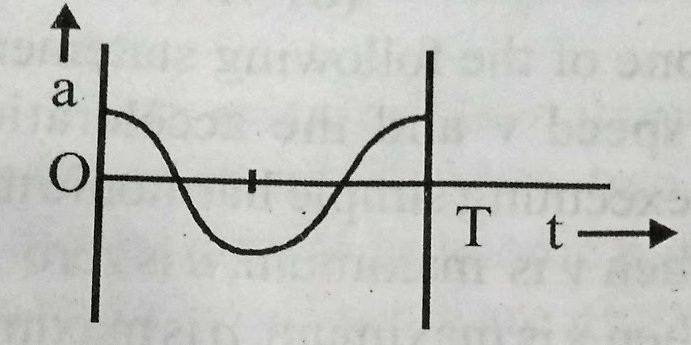• Option 2)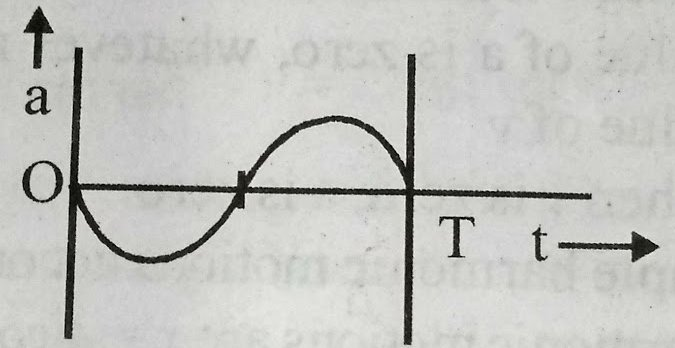• Option 3)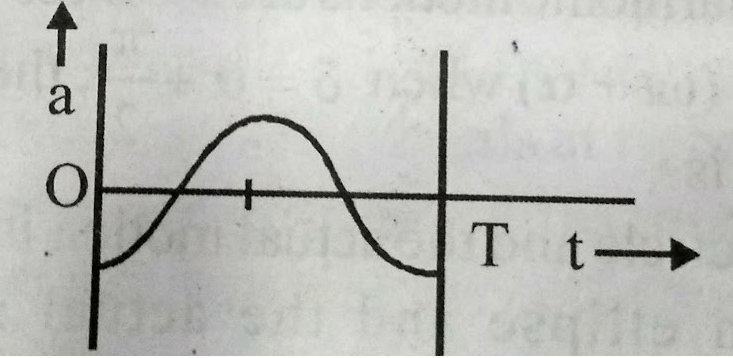• Option 4)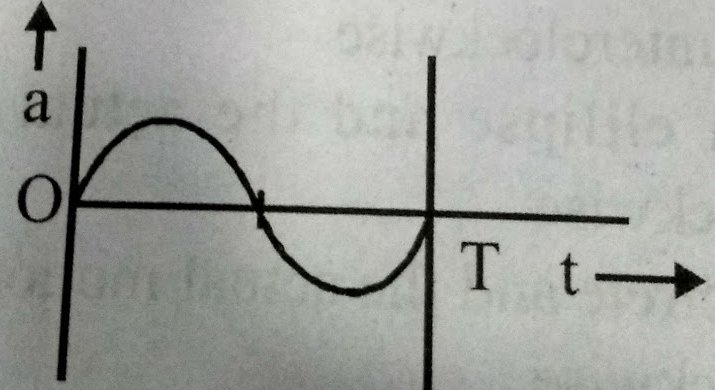As discussed Composition of two SHM in perpendicular direction -     - wherein Resultant equation    a=A coswt v=-AwSinwt a= i.e the variation of a with and correctly shown by graph (3) Option 1) This option is incorrect  Option 2) This option is incorrect  Option 3) This option is correct  Option 4) This option is incorrect
Medical
88 Views   |

The number of possible natural oscillation of air column in a pipe closed at one end of length 85 cm whose frequencies lie below 1250 Hz are:

( velocity of sound = 340 ms-1)

• Option 1)

4

• Option 2)

5

• Option 3)

7

• Option 4)

6

As learnt  Closed organ pipe - A closed organ pipe is a cylindrical tube having an air colume with one end closed. - wherein Condition of constructive interference         f =  if    n = 0     f0 = 100 Hz      n = 1      f1 = 300 Hz      n = 2      f2 = 500 Hz      n = 3      f3 = 700 Hz      n = 4      f4 = 900 Hz      n = 5      f5 = 1100 Hz      n = 6      f6 = 1300 Hz Possible natural...
Medical
71 Views   |

The displacement of a particle along the x-axis is given by x = a sin2t. The motion of the particle corresponds to:

• Option 1)

simple harmonic motion of frequency /

• Option 2)

simple harmonic motion of frequency 3/2

• Option 3)

non simple harmonic motion

• Option 4)

simple harmonic motion of frequency /2

As we discussed Composition of two SHM in perpendicular direction -     - wherein Resultant equation     This represent as s.n.m of frequency = w/ Option 1) simple harmonic motion of frequency / Correct Option 2) simple harmonic motion of frequency 3/2 Incorrect Option 3) non simple harmonic motion Incorrect Option 4) simple harmonic motion of frequency /2 Incorrect
Medical
132 Views   |

Sound waves travel at 350 m/s through a warm air and at 3500 m/s through a warm air and at 3500 m/s through brass. The wavelength of a 700 Hz acoustic wave as it enters brass from warm air

• Option 1)

decreases by a factor 10

• Option 2)

increases by a factor 20

• Option 3)

increases by a factor 10

• Option 4)

decreases by a factor 20

As we know Effect of frequency on speed of sound - With change in frequency of sound wave, wavelength also changes so that - wherein Thus, the speed of sound is independent of its frequency.     => Option 1) decreases by a factor 10 Incorrect Option 2) increases by a factor 20 Incorrect Option 3) increases by a factor 10 Correct Option 4) decreases by a factor 20 Incorrect
Medical
723 Views   |

A particle of mass m is released from rest and follows a parabolic path as shown. Assuming that the displacement of the mass from the origin is small, which graph correctly depicts the position of the particle as a function of time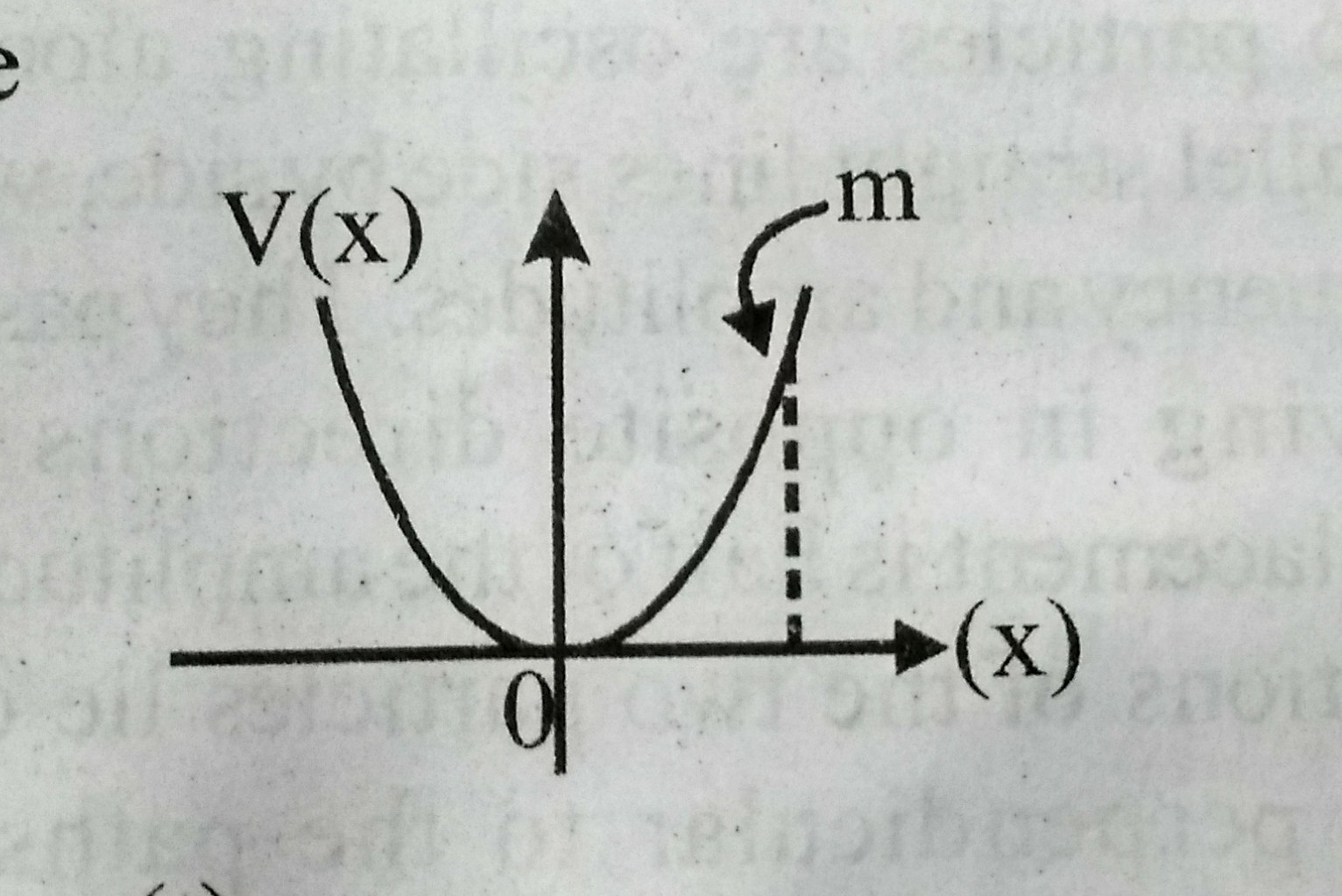• Option 1)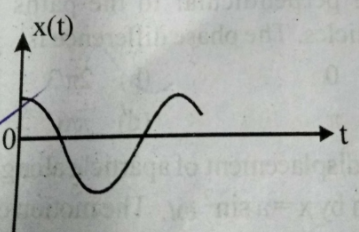• Option 2)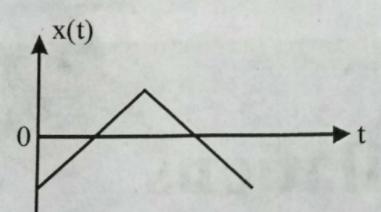• Option 3)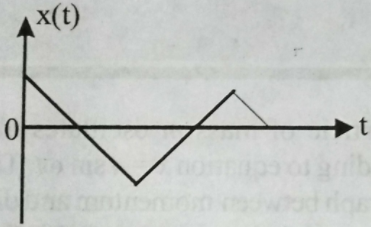• Option 4)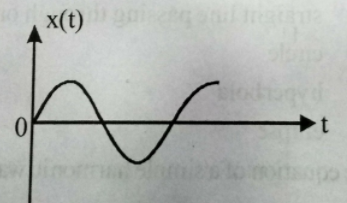Option 1) Option 2) Option 3) Option 4)
Exams
Articles
Questions